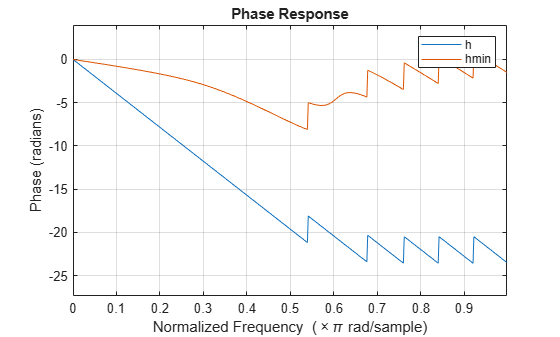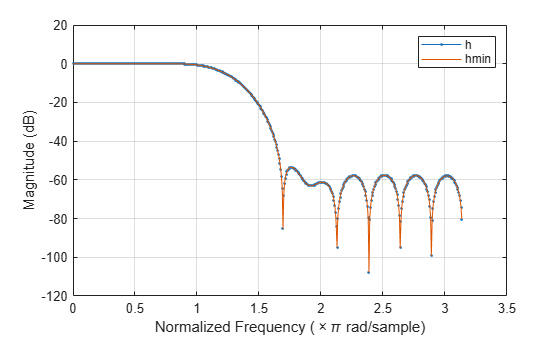Documentation

# polystab

Stabilize polynomial

## Syntax

```b = polystab(a) ```

## Description

`polystab` stabilizes a polynomial with respect to the unit circle; it reflects roots with magnitudes greater than 1 inside the unit circle.

`b = polystab(a)` returns a row vector `b` containing the stabilized polynomial. `a` is a vector of polynomial coefficients, normally in the z-domain:

`$A\left(z\right)=a\left(1\right)+a\left(2\right){z}^{-1}+\dots +a\left(m+1\right){z}^{-m}.$`

## Examples

collapse all

Use the window method to design a 25th-oder FIR filter with normalized cutoff frequency $0.4\pi$ rad/sample. Verify that it has linear phase but not minimum phase.

```h = fir1(25,0.4); h_linphase = islinphase(h)```
```h_linphase = logical 1 ```
`h_minphase = isminphase(h)`
```h_minphase = logical 0 ```

Use `polystab` to convert the linear-phase filter into a minimum-phase filter. Plot the phase responses of the filters.

```hmin = polystab(h)*norm(h)/norm(polystab(h)); hmin_linphase = islinphase(hmin)```
```hmin_linphase = logical 0 ```
`hmin_minphase = isminphase(hmin)`
```hmin_minphase = logical 1 ```
```hfvt = fvtool(h,1,hmin,1,'Analysis','phase'); legend(hfvt,'h','hmin')```Verify that the two filters have identical magnitude responses.

```hfvt = fvtool(h,1,hmin,1); legend(hfvt,'h','hmin')```## Algorithms

`polystab` finds the roots of the polynomial and maps those roots found outside the unit circle to the inside of the unit circle:

```v = roots(a); vs = 0.5*(sign(abs(v)-1)+1); v = (1-vs).*v + vs./conj(v); b = a(1)*poly(v); ```